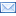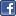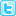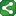Thursday, November 4, 2010

MDY Function

The MDY function converts MONTH, DAY, and YEAR values to a SAS date value. For example, MDY(10,19,1999) returns the SAS date value '19OCT99'D.

Syntax:    MDY(month,day,year)

Arguments

month : specifies a numeric expression that represents an integer from 1 through 12.

day    :specifies a numeric expression that represents an integer from 1 through 31.

year   :specifies a two-digit or four-digit integer that represents the year. The YEARCUTOFF= system    option defines the year value for two-digit dates.

If you know month, day, and year values, it’s very easy to derive date variable. You just need to use MDY function (of course, month, day, and year should be numeric). However, if the data is character then the conversion to numeric should occur first and then the conversion to the date value.

Example:

*When month, day, and year has numeric values;
data test;
year=1999;
month=12;
day=19;
newdate=mdy(month,day,year);
format newdate yymmdd10.;
run;

*When month, day, and year has character values;
data test;
year='1999';
month='12';
day='19';
newdate=mdy(input(month,2.),input(day,2.),input(year,4.);
format newdate yymmdd10.;
run;

You can use CATX and Input functions to get the same result.

newdate=input(catx('-',year,month,day),yymmdd10.);

Ref: SAS Help and Documentation.ShareThis